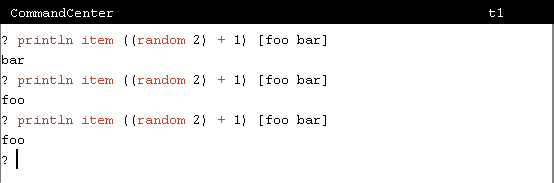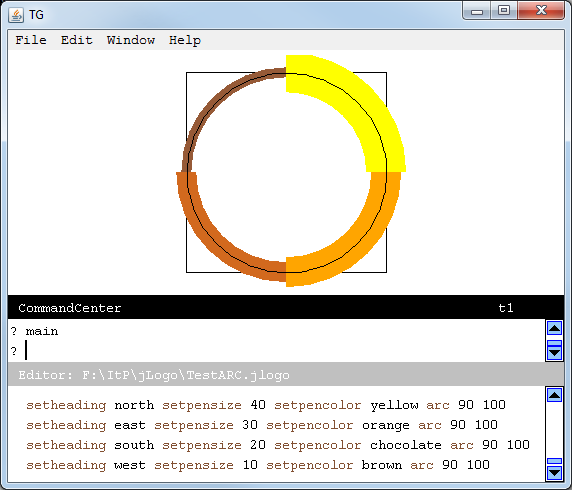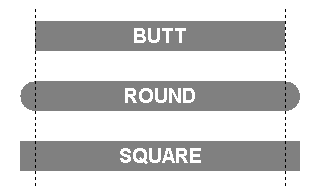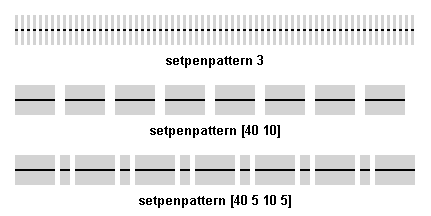BFOIT - Introduction to Computer Programming

# New TG v.9.38, v.9.38.1 Features - January 18, 2016

## Introduction

Version .0.9.38 of TG has support for the symbolic operators: *, +, -, /, <, =, and >.  They may be used in both an infix and prefix manner.

Version .0.9.38.1 improves graphics support. New commands (ARC, SETLINECAP, SETPENPATTERN) provide additional capabilities. Line drawing, area fill, and pixel manipulation are much faster. Lines drawn look a bit better.

## Symbolic Operators

The most annoying restriction in TG's Logo interpreter has been that its math operations have only been available as named procedures: DIFFERENCE, PRODUCT, QUOTIENT, and SUM.  I've wanted to use the symbolic characters '-', '*', '/', and '+' for a long time, but was afraid to because I thought they should be reserved for future use as infix operators, if I ever gave in and implemented them.  Basically, I wanted BFOIT students to learn about prefix notation - they would learn infix notation in a future programming language, Python, Java, C++, ...

TG's editor has no horizontal scroll bar.  Only a few years ago, it had no line continuation character (tilde, '~').  I did this to try to force students to write lots of small procedures.  It can be challenging to get students to break their programs into lots of little procedures.  What I found students doing was editing multi-line Logo commands and when complete, joining the lines together.  But this sometimes interfered with highlighting stuff when displaying error messages.  The stuff to highlight was off the display.  So, I gave in and added the line continuation character.

Now I've given in and added support for symbolic operators.  I've added them in such a way that they can be used in either a prefix or infix manner.  In version .9.38 of TG, a few single character symbols can be used instead of their equivilent named operators.

 * PRODUCT + SUM - DIFFERENCE / QUOTIENT < LESS? = EQUAL? > GREATER?

To use these symbolic operators in a prefix manner, place the complete operation in parenthesis.  As an example:

 `````` println product 256 256    `````` is equivilent to `````` println (* 256 256)    `````` `````` println quotient 65536 64    `````` is equivilent to `````` println (/ 65536 64)    `````` `````` if less? :foo 16 [stop]    `````` is equivilent to `````` if (< :foo 16) [stop]    ``````

## Infix Operators

Expressions of the form "100 + 2 * C6" are common in computer software.  You could enter this expression as a legal formula into a cell in a spreadsheet. Infix arithmetic operators and numerical conditionals have been included in Logo since its start.  So, version .9.38 of TG supports infix expressions involving a few single character symbols.  Here are the supported infix operators and their precedence.

 Symbol Precedence * 4 / 4 + 3 - 3 < 2 = 2 > 2

The precedence determines when operations get evaluated.  As this table shows, multiplication and division have a higher precedence than addition and subtraction.  So in an expression like

```      3 * 4 - 24 / 2
```

the multiplication and division operations will be evaluated before the subtraction operation.

```      3 * 4 - 24 / 2
12  -   12
0
```

Numerical comparisons have a lower precedence than the arithmetic operations.

```      6 + 4 = 24 / 2
6 + 4 =   12
12  =   12
true
```

Parenthesis can be used to override precedence.

```      (10 + 10) * 2
```

```      (10 + 10) * 2
20     * 2
40       ```

### Infix Operator Gotchas

There are a couple of things that you should be aware of when using infix symbolic operators.

1. The collection of procedure inputs has a lower precedence than any infix operator. Parenthesis should be used to resolve any issues.

Take for example that you want to pick a random word in a sentence.  In the simple case of a sentence with two words, you want to provide the ITEM operator with either 1 or 2.  The following image shows what happens if you use the infix '+' operator, thinking you are adding 1 to the value output by random 2.What has happened here is that "2 + 1" is evaluated first, outputing 3 and this becomes the input to random.  So, it outputs a value of 0, 1, or 2.  This is not what was intended.  The correct expression is: item ((random 2) + 1).2. When using '+' (plus) and '-' (minus) symbols as operators you must make sure they are not interpreted to be a sign being applied to a literal number. In these cases, follow them with at least one space.## New Commands

• The ARC command draws an arc of a circle, with the turtle at the center, with the specified radius, starting at the turtle's heading and extending clockwise through the specified angle. The turtle does not move.• The SETLINECAP command determines the appearance of the ends of lines drawn when the turtle moves with the pen down.  InputValue Type Description 0 BUTT Lines end where the turtle stops. This is the default. 1 ROUND Line endpoints are rounded with a semicircle. 2 SQUARE Line endpoints are extended one half of the pensize.• The SETPENPATTERN command provides the ability for the turtle to draw dashed lines. It takes either a number or a sentence of numbers as its input.

With an input of zero ('0') or an empty sentence ('[ ]'), the turtle draws a solid line.  Otherwise the turtle draws some amount then picks up the pen for some amount, repeating this sequence until it gets where it's going.  An input of [10 5] means ten turtle steps with the pen down followed by five steps with it up - this pattern repeated. If the input is a non-zero number, it is treated as identical up/down amounts.## New Operators

Two new operators (shortcuts) are available: GREATEREQUAL? an LESSEQUAL?. And the new PI operator outputs the ratio of a circle's circumference to its diameter.

 Name Input(s) Description GREATEREQUAL? GREATEREQUALP value1 value2 If value1 is greater than or equal to value2, GREATEREQUAL? outputs true.  If not, false is output. LESSEQUAL? LESSEQUALP value1 value2 If value1 is less than or equal to value2, LESSEQUAL? outputs true.  If not, false is output. PI Outputs the value 3.141592653589793.

## Finally...

Error messages associated with Arrays, Lists, and Words have been improved.  The source code associated with an error is now highlighted where previously it was not.

ARCTAN with two inputs, surrounded with parenthesis, e.g., (ARCTAN x y), outputs the arctangent of y/x, if x is nonzero, or 90 or –90 depending on the sign of y, if x is zero.

A few bugs have been fixed, including when a tilde ends a comment - it is now just part of the comment.

Checkout details at:This work (BFOIT: Introduction to Computer Programming, by Guy M. Haas),
identified by Berkeley Foundation for Opportunities in IT (BFOIT),
is free of known copyright restrictions.